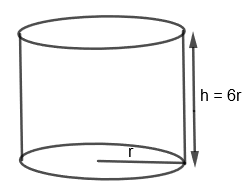Courses
Courses for Kids
Free study material
Free LIVE classes
More# The cost of painting the total outside surface of a closed cylindrical oil tank at 60 paise per sq. dm is Rs. 237.60. The height of the tank is 6 times the radius of the base of the tank. Find its volume correct to two decimal places.Verified
325.5k+ views
Hint: First of all find the outer surface by dividing the total cost of the painting by cost per sq. dm. Then use the formula of the total surface area of the cylinder that is $2\pi r\left( r+h \right)$ to find the radius and height of the tank. Use this radius and height to find the volume that is $\pi {{r}^{2}}h$.

We are given that the cost of painting the total outside surface of the closed cylindrical oil tank at 60 paise per sq. dm is Rs. 237.60. Also, the height of the tank is 6 times the radius of the base of the tank. We have to find the volume of the cylinder.
Let us consider the cylindrical tank diagrammatically.Let us consider the total outside surface area of the cylindrical tank as S $\text{d}{{\text{m}}^{2}}$.
We are given that the cost of painting per sq dm = 60 paise
So, we get the cost of painting the total outside surface area of the cylinder (S) = 60 S paise
We know that 1 paise $=\text{Rs}\text{.}\dfrac{1}{100}$
So, we get, 60 S paise $=\text{Rs}\text{.}\dfrac{60S}{100}$
Also, we are given that the total cost of the painting = Rs. 237.60
So, we get,
$\text{Rs}\text{.}\dfrac{60S}{100}=\text{Rs}.237.6$
By multiplying $\dfrac{100}{60}$ on both the sides, we get,
$S=\left( \dfrac{100}{60} \right)\times \left( 237.6 \right)$
$S=396\text{ }d{{m}^{2}}$
Now, we know that the surface area of the cylinder $=2\pi r\left( r+h \right)$ where r and h are the radius and height of the cylinder respectively.
We are given that the height is 6 times the radius of the base of the tank. So, we get,
h = 6r
So, we get the total outer surface area of the tank $=2\pi r\left( r+6r \right)$
\begin{align} & =2\pi r.7r \\ & =14\pi {{r}^{2}} \\ \end{align}
We have already found that the total outer surface area of the tank $=396\text{ d}{{\text{m}}^{2}}$. So, we get,
$14\pi {{r}^{2}}=396\text{ d}{{\text{m}}^{2}}$
Or, ${{r}^{2}}=\dfrac{396}{14\pi }$
By substituting $\pi =\dfrac{22}{7}$, we get,
${{r}^{2}}=\dfrac{396\times 7}{14\times 22}$
By simplifying the above equation, we get,
${{r}^{2}}=9$
By taking square root on both the sides, we get,
$r=\sqrt{9}=3\text{ dm}$
So, we get, h = 6r = 6 x 3 = 18 dm.
Now, we know that the volume of the cylinder $=\pi {{r}^{2}}h$
So, we also get the volume of the cylindrical tank $=\pi {{r}^{2}}h$
By substituting r = 3 dm and h = 18 dm, we get,
The volume of the cylindrical tank $=\left( \dfrac{22}{7} \right)\left( {{3}^{2}} \right)\left( 18 \right)$
$=\dfrac{22}{7}\times 9\times 18$
$=509.14\text{ d}{{\text{m}}^{3}}$
Hence, we get the volume of the cylindrical tank as $509.14\text{ d}{{\text{m}}^{3}}$.

Note: In this question, students often make mistakes in the conversion of units and sometimes even forget to convert one unit into another. So, these mistakes must be avoided and students should properly consider the units as well with magnitudes of each quantity. Also, students must remember the basic conversions like Re. 1 = 100 paise.
Last updated date: 30th May 2023
Total views: 325.5k
Views today: 5.82k